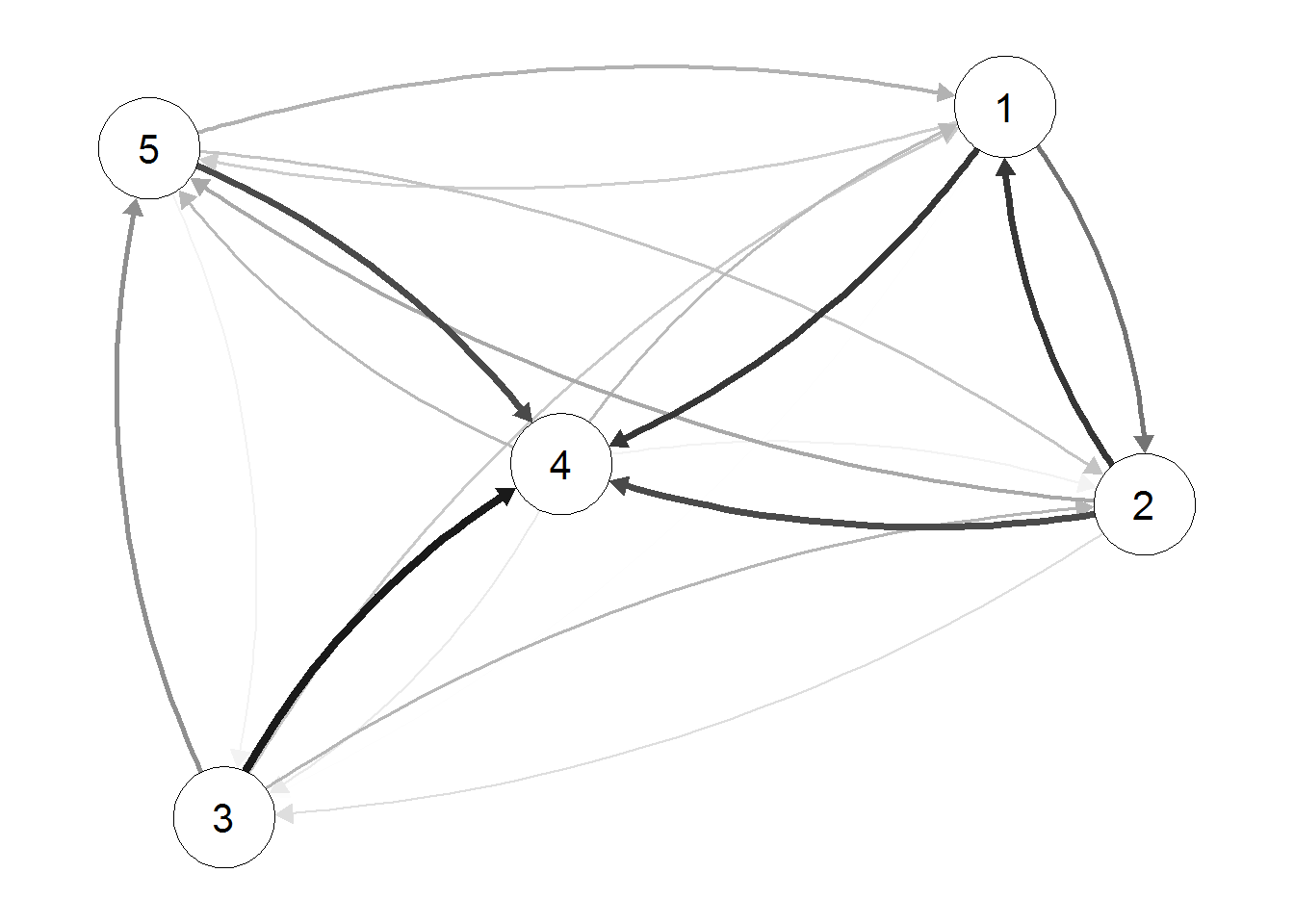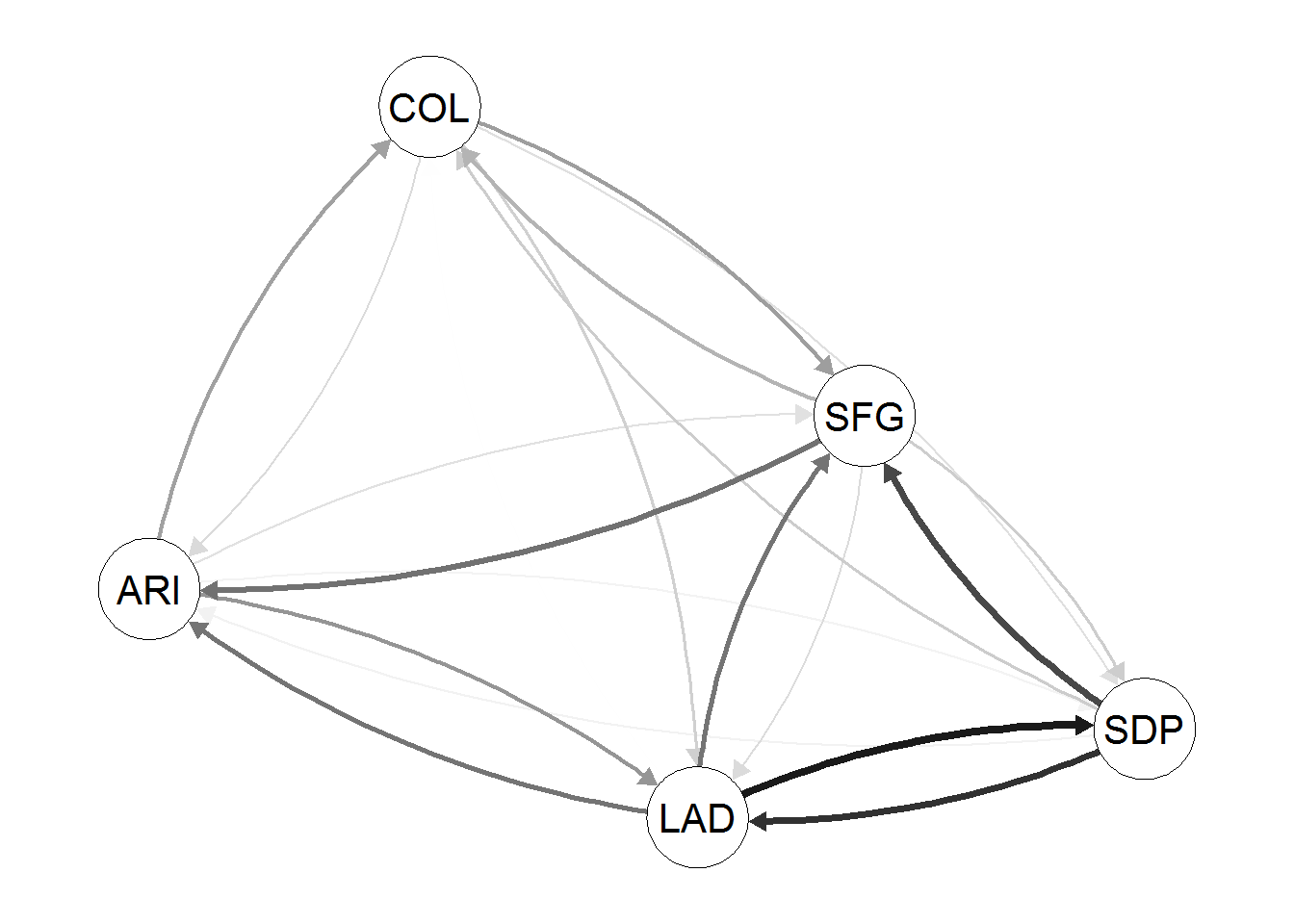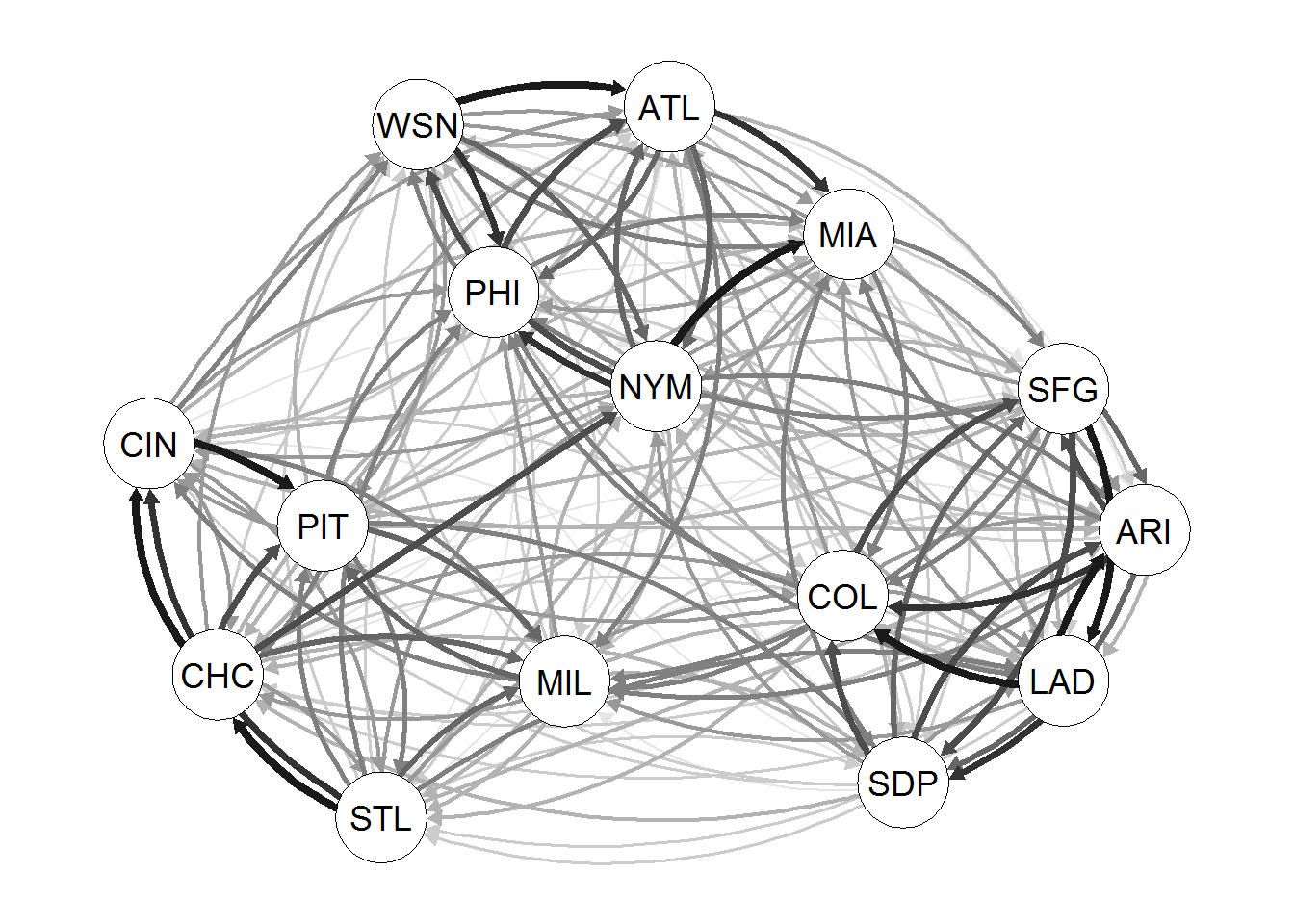I will attempt to plot a network showing which MLB (Major League Baseball) teams are currently winning their season series over other teams.

### A starter example

To test out the qgraph package, I will run an example found at: http://stackoverflow.com/questions/7521381/draw-network-in-r-control-edge-thickness-plus-non-overlapping-edges

Edges <- data.frame(
from = rep(1:5,each=5),
to = rep(1:5,times=5),
thickness = abs(rnorm(25)))

Edges <- subset(Edges,from!=to)

library("qgraph")
qgraph(Edges,esize=5,gray=TRUE)### Will this work with categorical nodes?

Next, I will try to insert baseball team names into the from and to variables.

Edges <- data.frame(
from = rep(c("ARI", "COL", "LAD", "SDP", "SFG"),each=5),
to = rep(c("ARI", "COL", "LAD", "SDP", "SFG"),times=5),
thickness = abs(rnorm(25)))
Edges <- subset(Edges,from!=to)

qgraph(Edges,esize=5,gray=TRUE)## The Data Set

I will analyze the National League head-to-head records as seen at http://www.baseball-reference.com/leagues/NL/2015-standings.shtml. This data for the current season was last updated on August 6, 2015.

mlb <- read_excel("MLB.xlsx")
rownames(mlb) <- c("ARI", "ATL", "CHC", "CIN", "COL", "LAD", "MIA", "MIL", "NYM", "PHI", "PIT", "SDP", "SFG", "STL", "WSN")
mlb[1:5, 1:5]
##     ARI ATL CHC CIN COL
## ARI   0   2   2   0   8
## ATL   1   0   1   3   0
## CHC   1   2   0   9   4
## CIN   0   4   4   0   2
## COL   4   4   2   4   0

## Making the Edge List

Using the igraph package, I next form a list of the network edges (for later use in the qgraph). Note the need for weighted = TRUE to bring the weights along.

library(igraph)
##
## Attaching package: 'igraph'
##
## The following objects are masked from 'package:stats':
##
##     decompose, spectrum
##
## The following object is masked from 'package:base':
##
##     union
A <- graph.adjacency(as.matrix(mlb), weighted = TRUE)
edges <- get.data.frame(A)
head(edges)
##   from  to weight
## 1  ARI ATL      2
## 2  ARI CHC      2
## 3  ARI COL      8
## 5  ARI MIA      5
## 6  ARI MIL      5

A quick inspection revealed that the zero-weights were dropped from the list (a desirable action).

## Plotting the Network

Finally, the qgraph command will draw a directed graph and emphasize—with darker arrows—the number of wins over another team.

qgraph(edges,esize=5,gray=TRUE)Since the adjacency matrix referred to the number of wins between pairs of teams, notice the clustering into the MLB divisions!